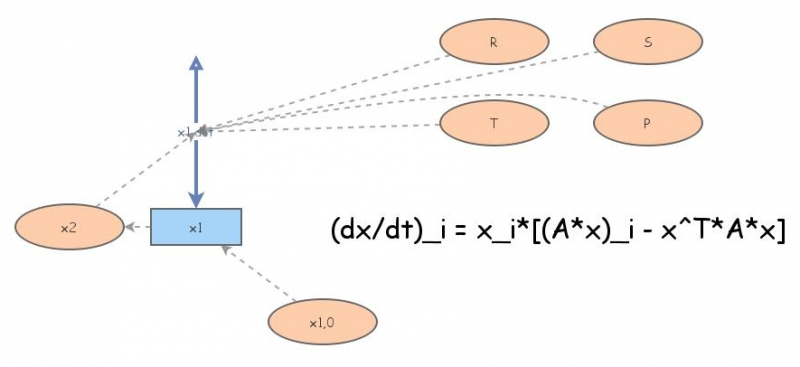Prisoner's dilemma with replicator equationSmall replicator equation setup (2d) with prisoner's dilemma payoff matrix (can be adjusted): (dx/dt)_i = x_i*((A*x)_i-x^T*A*x)